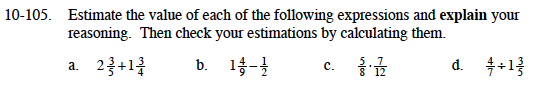### Home > MC1 > Chapter 10 > Lesson 10.2.3 > Problem10-105

10-105.$\text{Both }\frac{3}{5} \text{ and }\frac{3}{4} \text{ are a little over }\frac{1}{2} \text{ so the sum }$

$\text{should be a little above, but close to }4.$

$4\frac{7}{20}$

$\frac{4}{9} \text{ is a little less than \frac{1}{2} so the difference}$

$\text{should be a little less than 1.}$

$\frac{5}{8}\left(\frac{7}{12}\right)=\frac{35}{96}$

$\text{This is close to }\frac{1}{2}\div1\frac{1}{2}=\frac{1}{2}\div\frac{3}{2}=\frac{1}{3}$